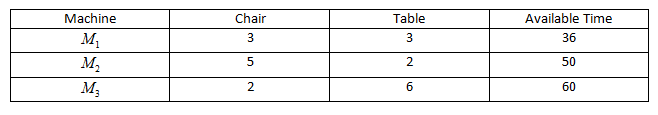Courses
Courses for Kids
Free study material
Free LIVE classes
MoreLIVE
Join Vedantu’s FREE Mastercalss

# A firm makes two types of furniture: chairs and tables. The contribution to the profit for each product as calculated by the accounting department is Rs. 20 per chair and Rs. 30 per table. Both products are to be processed on three machines ${M_1},{M_2}{\text{ and }}{M_{3.}}$ The time required in hours by each product and total time available in per hours week on each machine are as follows:Which manufacturer gained more profit?A.${M_1}$B.${M_2}$C.${M_3}$D.NoneVerified
358.2k+ views
Hint- To solve the above question, we need to first calculate total profit gained by each machine. This can be done by finding the number of chairs/tables manufactured per week and then calculating the profit of each machine.

For machine ${M_1}$
From the table three chairs are manufactured per hour
Total time available per week =36
Total chair possible in one week
$= \dfrac{{36}}{3} = 12$
Total table manufacture per hour=3
Total table possible in one week
$= \dfrac{{36}}{3} = 12$
Therefore, the total profit per week is
$= 12 \times 20 + 12 \times 30 \\ = Rs.600 \\$

For machine ${M_2}$
From the table, five chairs are manufactured per hour
Total time available per week =50
Total chair possible in one week
$= \dfrac{{50}}{5} = 10$
Total table manufacture per hour=2
Total table possible in one week
$= \dfrac{{50}}{2} = 25$
Therefore, the total profit per week is
$= 10 \times 20 + 25 \times 30 \\ = Rs.950 \\$
For machine ${M_3}$
From the table two chairs are manufactured per hour
Total time available per week =60
Total chair possible in one week
$= \dfrac{{60}}{2} = 30$
Total table manufacture per hour=6
Total table possible in one week
$= \dfrac{{60}}{6} = 10$
Therefore, the total profit per week is
$= 30 \times 20 + 10 \times 30 \\ = Rs.900 \\$
Hence, the manufacturer used ${M_2}$ for production to maximize profit.

Note- These types of questions are statement based questions. In these types of questions read the statement carefully and gather all the information to form the given equation. After that, we start solving, in this question we have to calculate the profit of each machine per week. The maximum profit produced by the machine is our answer.
Last updated date: 21st Sep 2023
Total views: 358.2k
Views today: 10.58k